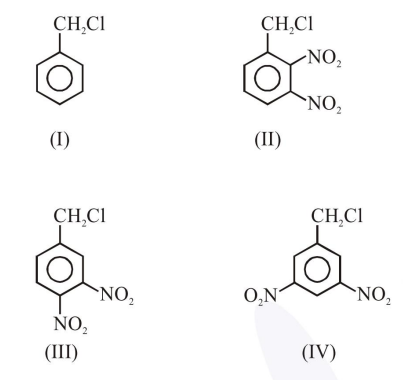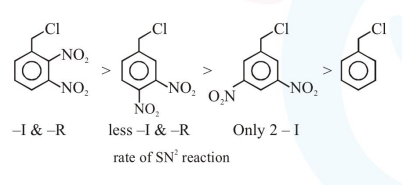# The decreasing order of reactivity of the following compounds towards nucleophilic

Question:

The decreasing order of reactivity of the following compounds towards nucleophilic substitution $\left(\mathrm{S}_{\mathrm{N}}^{2}\right)$ is :1. $(\mathrm{IV})>(\mathrm{II})>(\mathrm{III})>(\mathrm{I})$

2. (II) $>$ (III) $>$ (IV) $>$ (I)

3. (II) $>$ (III) $>$ (I) $>$ (IV)

4. (III) $>$ (II) $>$ (IV) $>$ (I)

Correct Option: , 2

Solution: